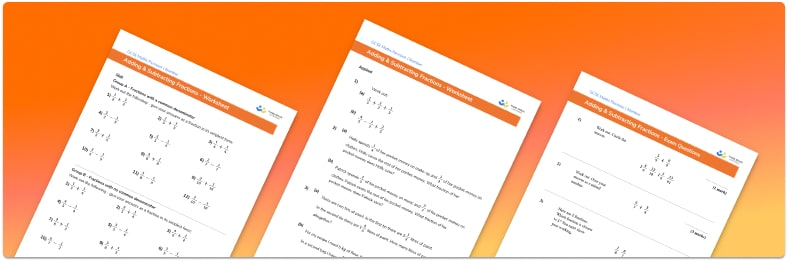# Add And Subtract Fractions Worksheet• Section 1 of the Adding and Subtracting Fractions worksheet contains 12 skills-based Adding and Subtracting Fractions questions, in 3 groups to support differentiation
• Section 2 contains 3 applied Adding and Subtracting Fractions questions with a mix of worded problems and deeper problem solving questions
• Section 3 contains 3 foundation and higher level GCSE exam style Adding and Subtracting Fractions questions
• Answers and a mark scheme for all Adding and Subtracting Fractions questions
• Follows variation theory with plenty of opportunities for students to work independently at their own level
• All questions created by fully qualified expert secondary maths teachers
• Suitable for GCSE maths revision for AQA, OCR and Edexcel exam boards

• This field is for validation purposes and should be left unchanged.

You can unsubscribe at any time (each email we send will contain an easy way to unsubscribe). To find out more about how we use your data, see our privacy policy.

### Adding and subtracting fractions at a glance

Fractions can only be added or subtracted if they have like denominators – or in other words, if they are like fractions. If fractions already have the same denominator, we simply add or subtract the numerators as required.

#### How to add and subtract fractions

If the fractions have different denominators (unlike fractions), we use knowledge of equivalent fractions to change one or both denominators – this is called finding a common denominator. For example, if one fraction had a denominator of 4, and the other had a denominator of 6, we could change both fractions to have denominator 12, as 12 is the LCM (lowest common multiple) of 4 and 6. Once both fractions have been written with a common denominator we add or subtract the numerators as required.

Fraction addition and subtraction of fractions can be extended to working with mixed numbers (sometimes called mixed fractions); a mixed number has both a whole number part and a simple fraction part. We can convert between mixed numbers and improper fractions in order to calculate with them.

Looking forward, students can then progress to additional number worksheets, for example aor aFor more teaching and learning support on Number , our GCSE maths lessons provide step by step support for all GCSE maths concepts.

## Do you have KS4 students who need more focused attention to succeed at GCSE?There will be students in your class who require individual attention to help them succeed in their maths GCSEs. In a class of 30, it’s not always easy to provide.

Help your students feel confident with exam-style questions and the strategies they’ll need to answer them correctly with our dedicated GCSE maths revision programme.

Lessons are selected to provide support where each student needs it most, and specially-trained GCSE maths tutors adapt the pitch and pace of each lesson. This ensures a personalised revision programme that raises grades and boosts confidence.

Find out more